# historical word embeddings & lexical semantic change

I have developed a Git Hub guide that demonstrates a simple workflow for sampling Google n-gram data and building historical word embeddings with the aim of investigating lexical semantic change. Here, we build on this workflow, and unpack some methods presented in Hamilton, Leskovec, and Jurafsky (2016) & Li et al. (2019) for aligning historical matrices/embeddings and visualizing semantic change.

library(tidyverse)

As detailed in the Git Hub post, the historical embeddings utilized here span eight quarter-centuries from 1808 to 2008, and are derived from a ~1% sample of Google’s English One Million 5-gram corpus. Long story short of workflow:

• Build term-feature matrices based on simple co-occurrence;
• Weight/transform matrices via positive-pointwise mutual information;
• Homogenize feature set across all time periods;
• Compress feature set to 250 latent dimensions via SVD.

The count of terms included in each quarter-century varies from ~16k to ~18k. Matrices are available on Git Hub here.

setwd(local_dir)
tfms_mats <- readRDS('tfms_mats.rds')

For subsequent analyses, we reduce matrices to only terms which occur in every quarter-century, which amounts to roughly 11k forms.

all_terms <-Reduce(intersect,
lapply(tfms_mats, rownames))

tfms_trimmed <- lapply(1:8, function (x)
tfms_mats[[x]][rownames(tfms_mats[[x]]) %in% all_terms,])
names(tfms_trimmed) <- names(tfms_mats)

Frequency data for all terms included in the historical matrices are available here. These frequencies are based on the sampled 5-gram corpus, and will obviously differ some from values obtained from the full corpus.

setwd(local_freq)
freqs_by_gen <- readRDS('freqs_by_gen.rds')

While we are here, we examine historical frequencies for a small set of forms.

sample <- c('GRASP', 'SIGNIFICANT', 'COMMITMENT', 'HOPE')

freqs_by_gen %>%
filter(form %in% sample) %>%

ggplot(aes(x = quarter, y = ppm,
color = form, group = form)) +
geom_line(size = 1.5) +
ggthemes::scale_color_stata()+
facet_wrap(~form, scales = 'free_y', ncol=2) +
labs(title="Historical frequencies of some forms on the move") +
theme(legend.position="none",
axis.text.x=element_text(angle=45,
hjust=0.5, vjust=0.5))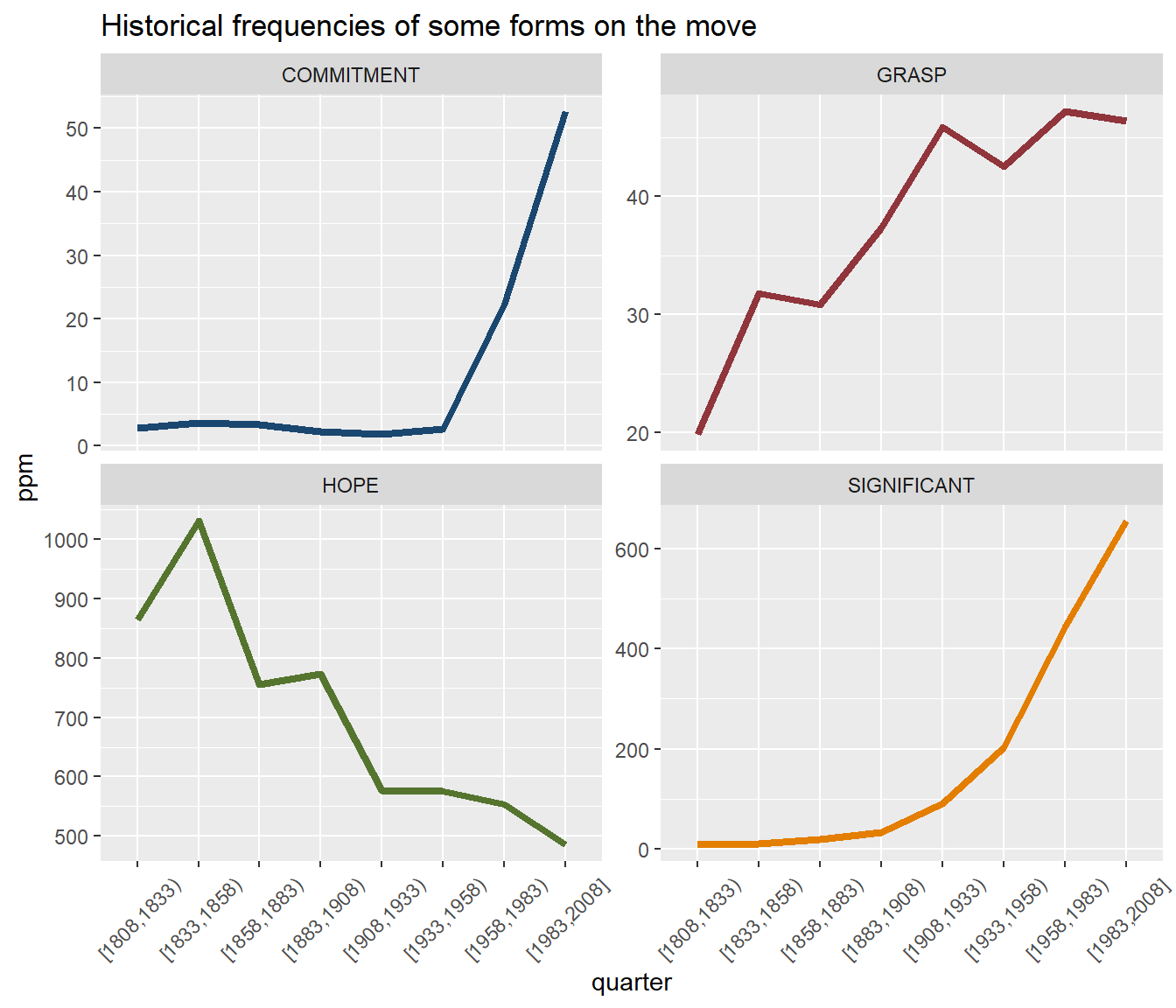## Nearest-neighbors

Based on these historical matrices, we first investigate historical nearest-neighbors/synonyms for a given term. Using the neighbors function from the LSAfun package, we extract the top 10 nearest-neighbors for each quarter-century for the form SIGNIFICANT.

search <- toupper('significant')
nns <- lapply(tfms_trimmed, LSAfun::neighbors, x = search , n = 10)
#disability, disorder, CELL, quit, significant, 

List output from above is a bit messy; the function below cleans this output some for subsequent visualization/analyses.

strip_syns <- function (x) {
lapply(1:length(x), function(y)
cbind(form = as.character(names(x[[y]])),
quarter = names(x[y]),
data.frame(sim = x[[y]], row.names=NULL)) ) %>%
bind_rows()}

We apply function, and add historical frequency data to get a better sense of how changes in nearest-neighbors align (or do not align) with changes in term frequency historically.

syns <- nns %>%
strip_syns() %>%
inner_join(freqs_by_gen) %>%
mutate(ppm = round(ppm, 1), sim = round(sim,3)) %>%
select(-freq) %>%
group_by(quarter) %>%
arrange(desc(sim))%>%
ungroup()

Via gridExtra, we plot nearest-neighbors of SIGNIFICANT by quarter-century.

g <- list(length(tfms_mats))
tt <- gridExtra::ttheme_default(base_size = 6.75)

for (i in 1:length(tfms_mats)) {
g[[i]] <- syns %>%
filter (quarter == names(tfms_mats[i])) %>%
rename(!!names(tfms_mats[i]) := form) %>%
select(-quarter)%>%
gridExtra::tableGrob(rows=NULL, theme = tt) }

gridExtra::grid.arrange(grobs = g, nrow = 2)So, a form on the semantic move historically. Roughly: CONSPICUOUS/ REMARKABLESUBSTANTIAL/ MARKED. Also a form that has become decidedly more frequent. Collectively, changes (very roughly) reflect (1) the (novel) use of SIGNIFICANT in the (burgeoning, ca. late 19th century) field of Statistics, and (2) the continued development of the field of Statistics over the past century or so.

## Procrustes, PCA & visualizing semantic change

While each of our historical term-feature matrices is comprised of 250 latent dimensions, per the nature of SVD this space is defined differently for each matrix. And while this is non-problematic when computing pair-wise cosine similarities within a given time period (as per above), it is problematic if the goal is to compare word embeddings for a single form across multiple time periods. Which is what we want to do now.

In order to perform this type of comparison, we need to map each historical matrix to a singularly defined coordinate space. Here, this means aligning all non-modern matrices to the modern matrix. We do so using orthogonal Procrustes, and implement the algorithm using the procrustes function from the vegan package.

procrustes_clean <- lapply(1:7, function (x) {

pc <- vegan::procrustes (X = tfms_trimmed[],
Y = tfms_trimmed[[x]])
pc <- pc$Yrot dimnames(pc) <-list(rownames(tfms_trimmed[]), c(1:250)) pc } ) procrustes_clean[] <- tfms_trimmed[] names(procrustes_clean) <- names(tfms_mats) With compare-able matrices in tow, our first goal is to visualize semantic change for a given term in two-dimensional space. General workflow: 1. Find (some subset of) historical/modern nearest-neighbors for a given term; 2. Extract embeddings for (1) from modern matrix; 3. Extract embeddings for given term from all historical matrices; 4. Append (2) & (3) as single matrix; 5. Perform Principal Component Analysis (PCA) on (4); 6. Plot (5) in 2D space This workflow (roughly) mirrors methods presented in Li et al. (2019), which is a slightly simplified/modified version of methods presented in Hamilton, Leskovec, and Jurafsky (2016). What are we actually doing? Well, we are basically defining a 2d space via modern word embeddings of nearest-neighbors, and allowing a given term (via historical word embeddings) to move within this (defined) space historically. Procrustes-enabled: all forms in all matrices are situated in the same coordinate space. Obviously this is a bit weird, as nearest-neighbors are in motion within this space historically as well. However, we have to hold something constant to visualize change – modern embeddings for nearest-neighbors would seem most intuitive/insightful. To de-clutter our (forthcoming) visualizations some, we limit our analysis to even-numbered quarter-centuries. gens <- c("[1833,1858)", "[1883,1908)", "[1933,1958)", "[1983,2008]") 1. Find (some subset of) historical/modern nearest-neighbors for a given term; plot_syns <- syns %>% filter(!form %in% search & quarter %in% gens) %>% group_by(quarter) %>% slice(1:15) %>% ungroup() %>% select(form) %>% distinct() 2. Extract embeddings for (1) from modern matrix; syn_modern <- tfms_trimmed[][rownames(tfms_trimmed[]) %in% plot_syns$form,]

3. Extract embeddings for given term from all historical matrices;

term_proc <- lapply(procrustes_clean[c(2,4,6,8)],
function (x) x[search,]) %>%
bind_rows() %>% t()

4. Append (2) & (3) as single matrix;

full <- rbind(term_proc, syn_modern)

5. Perform Principal Component Analysis (PCA) on (4);

pca <- prcomp(full)$x[,1:2] %>% data.frame() %>% rownames_to_column() %>% mutate(cs = ifelse(grepl('\\[', rowname), 'steelblue', 'black')) %>% mutate(sz = ifelse(cs == 'steelblue', 3.5, 2.5)) 6. Plot (5) in 2D space. set.seed(99) #Hack to build arrows in viz. to_end <- pca %>% slice(1:4) for (i in 1:(nrow(to_end)-1)) { to_end$PC3[i] <- to_end$PC1[i+1] to_end$PC4[i] <- to_end$PC2[i+1] } ggplot(pca, aes(x=PC1, y=PC2))+ geom_point(color = 'darkgray', size = 1)+ ggrepel::geom_text_repel( data = pca, label = pca$rowname,
color = pca$cs, segment.size = .25, direction = "y", hjust = 0, size = pca$sz,
segment.alpha = .25) +
geom_segment(data = to_end %>% slice(1:3),
aes(x=PC1, y=PC2, xend =PC3, yend = PC4),
color = 'steelblue',
size = .6, linetype = 2,
arrow = arrow(length=unit(0.30,"cm"),
type = "closed",
angle = 25))+

ggthemes::theme_fivethirtyeight()  +
ggtitle(paste0(search, ' in nearest-neighbor space historically')) +
theme(axis.title.x=element_blank(),
axis.text.x=element_blank(),
axis.title.y=element_blank(),
axis.text.y=element_blank(),
plot.title = element_text(size=12))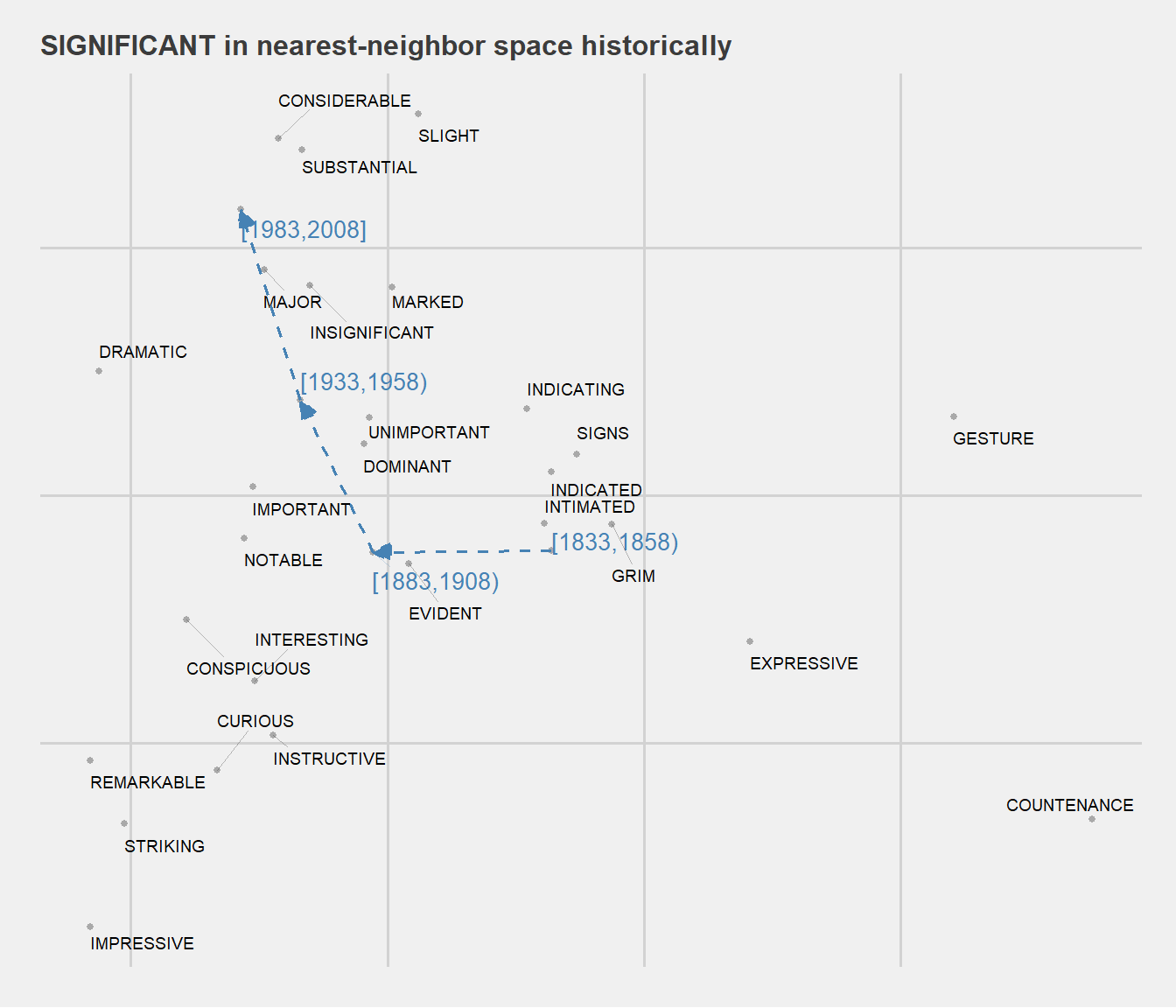For good measure, we consider another example: GRASP. Historical nearest-neighbor space is visualized below. Very generally, physical(ly) GRASPmental(ly) GRASP. By virtue of having a non-lemmatized cache of word embeddings, lots of fun details.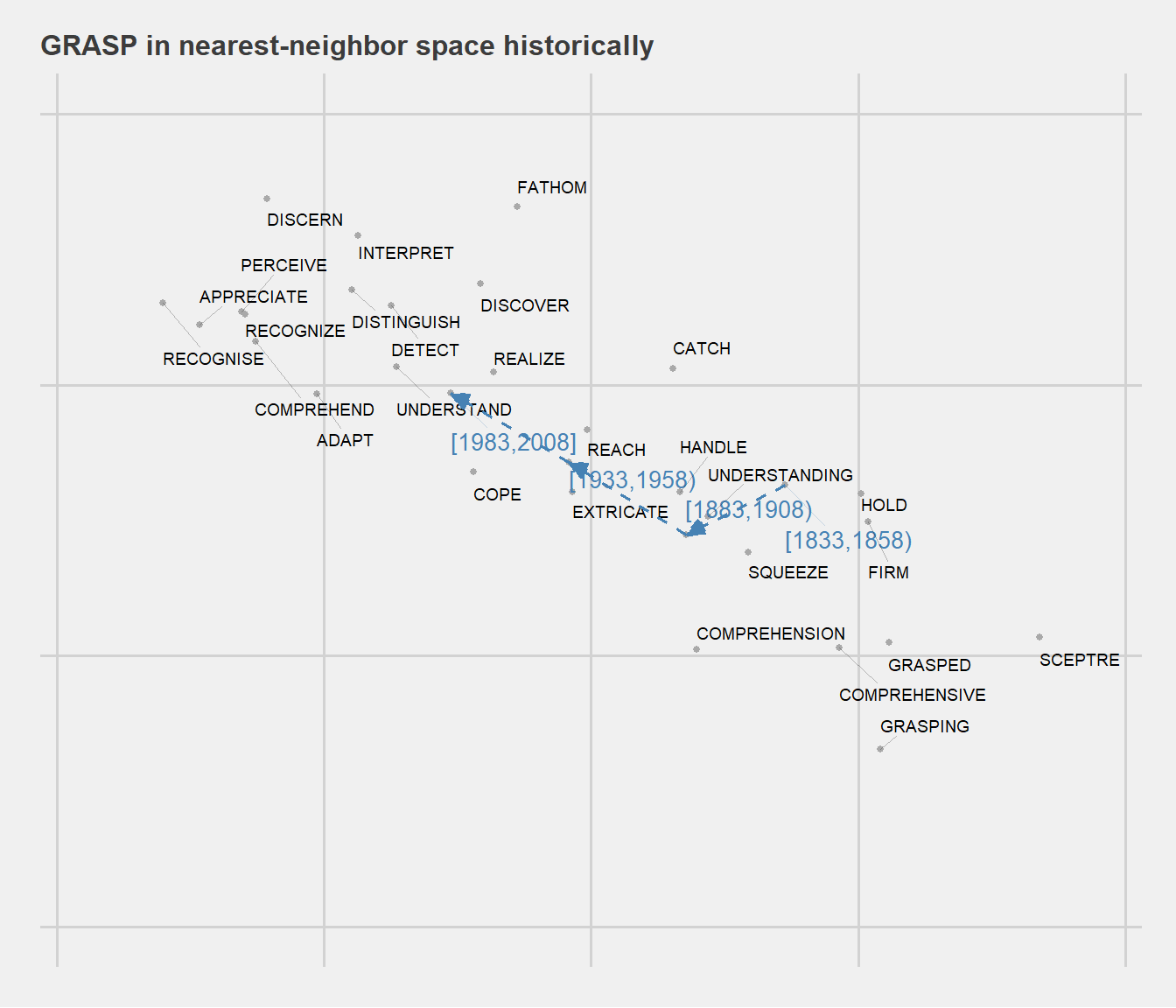## Detecting semantic change

Per Hamilton, Leskovec, and Jurafsky (2016), we can also compare a single form’s embeddings at two (or more) points in time to detect changes in distribution and, in theory, lexical semantics. Hamilton, Leskovec, and Jurafsky (2016) dub such changes semantic displacement – below we compute the degree of semantic displacement (as cosine distances) for all forms in our historical lexicon between quarter-centuries [1883,1908) and [1983,2008].

sim_deltas <- sapply(rownames(procrustes_clean[]), function (x)
lsa::cosine(procrustes_clean[["[1883,1908)"]][x,],
procrustes_clean[["[1983,2008]"]][x,]) ) %>%
#Clean --
as.tibble() %>%
rownames_to_column(var = 'form') %>%
mutate(value = round(value, 3)) %>%
arrange(desc(value)) %>%
rename(sim_t1_t2 = value)

The table below highlights forms with the highest degree of semantic displacement from [1883,1908) to [1983,2008]. Again, we add some frequency data to fill out the story some.

freq_deltas <- freqs_by_gen %>%
filter(quarter %in% c("[1883,1908)", "[1983,2008]") &
form %in% rownames(procrustes_clean[])) %>%
select(-freq) %>%
mutate(log_freq_t1_t2 = round(log([1983,2008]/[1883,1908)),3)) %>%
inner_join(sim_deltas)

freq_deltas %>% arrange(sim_t1_t2) %>% slice(1:6) %>% knitr::kable()
form [1883,1908) [1983,2008] log_freq_t1_t2 sim_t1_t2
MID 2.50 25.54 2.324 0.081
EXPLOIT 3.20 12.13 1.333 0.086
PITT 7.61 5.09 -0.402 0.113
LURE 2.00 4.36 0.779 0.114
SICKLE 1.98 4.91 0.908 0.120
COMMITMENT 2.32 52.74 3.124 0.123

Based on these findings, we take a quick look at COMMITMENT in nearest-neighbor space historically.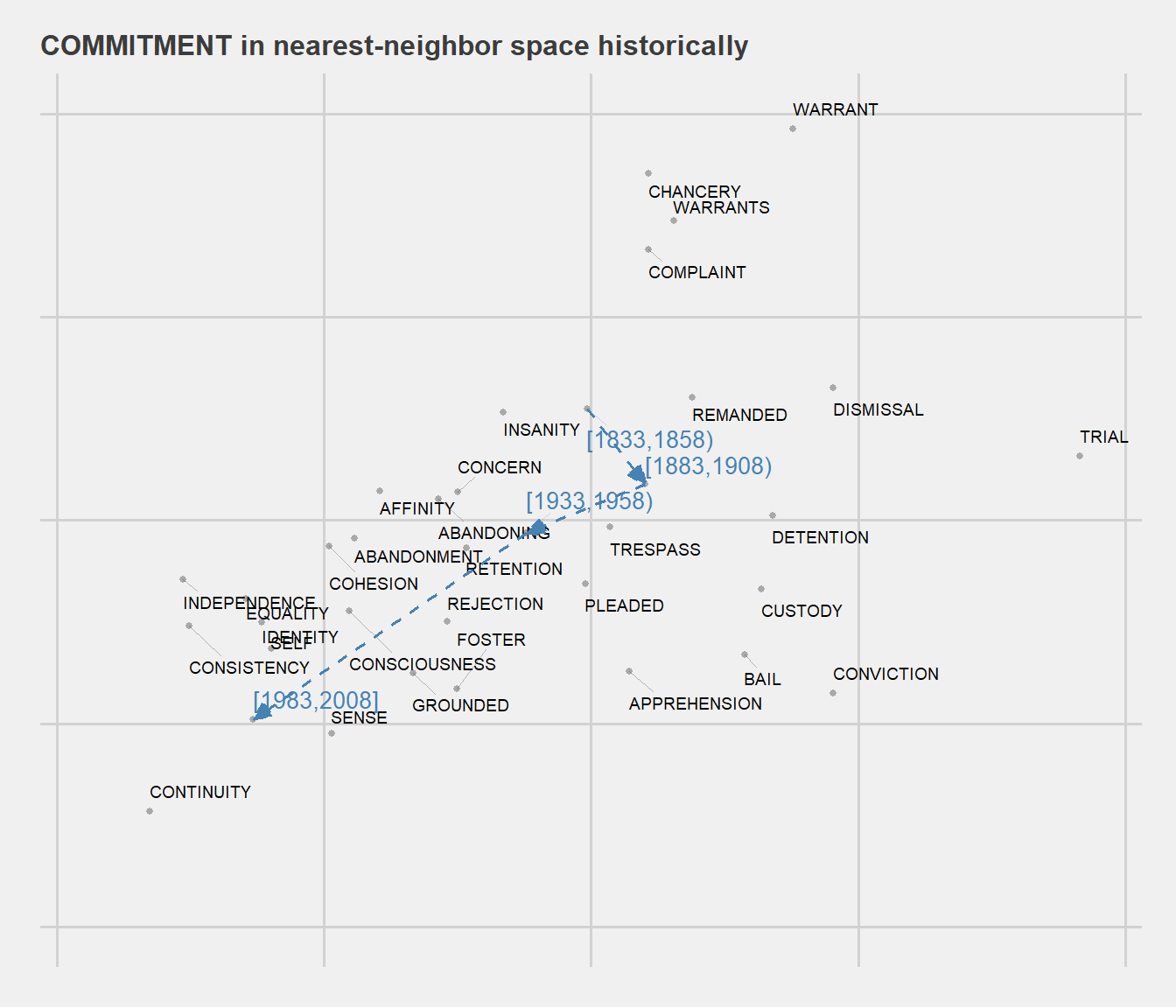The plot below illustrates the relationship between delta frequency and semantic displacement from t1 to t2 for all forms included in our lexicon. So, increases in frequency from t1 to t2 are generally accompanied by higher degrees of semantic displacement (ie, lower cosine similarity). Does novel use cause increases in frequency, or vice versa?

freq_deltas %>%
ggplot(aes(x = log_freq_t1_t2, y =sim_t1_t2)) +
geom_point(size = .25)+
geom_smooth(method="loess", se=T, color = 'steelblue')+
ggtitle('Delta frequency & semantic dispalcement: [1883,1908) to [1983,2008]')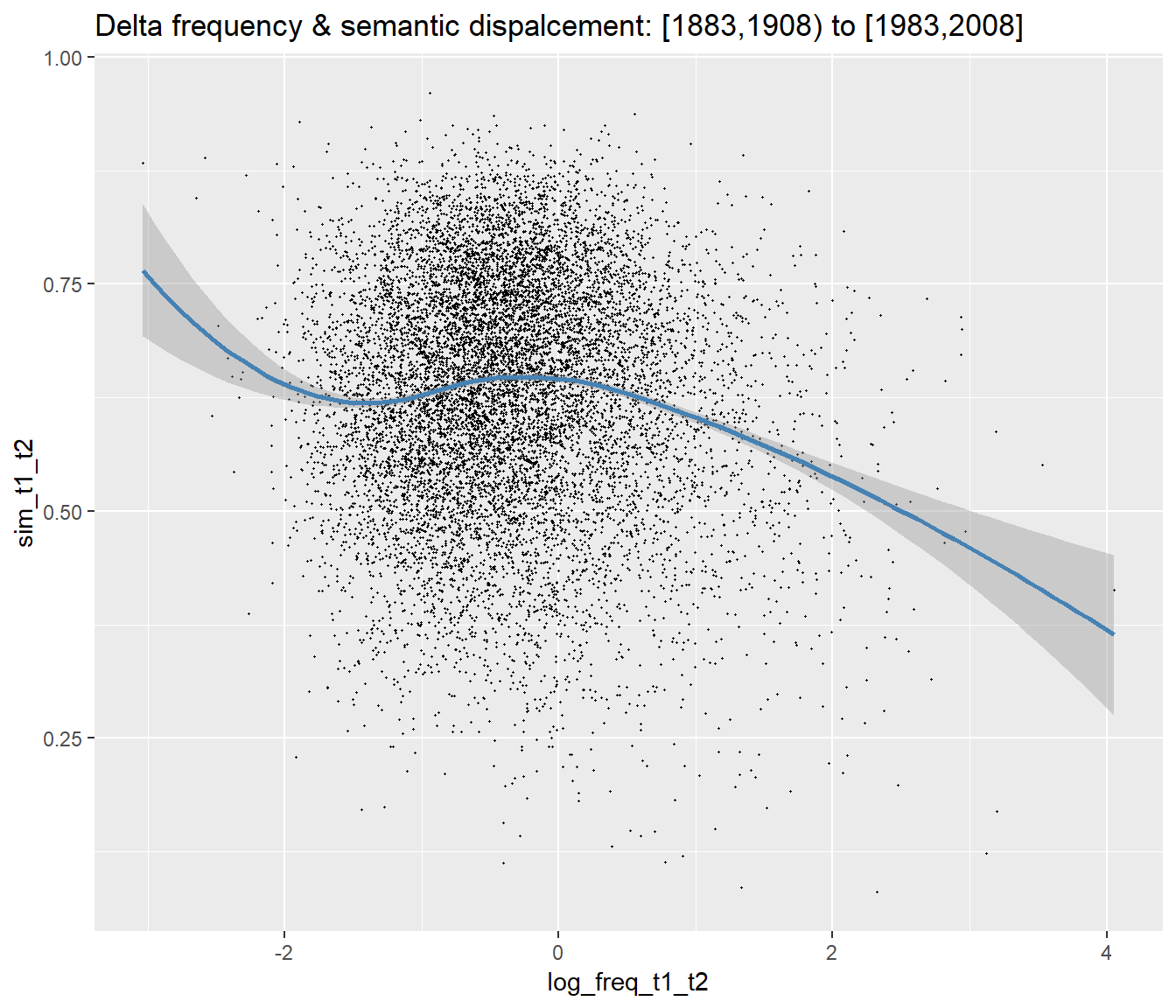## Summary

Grains of salt abound. These methods are imperfect, and I think best suited for exploration. Examples of actual usage always tell a more complete story when investigating lexical change. Also, the word embeddings used here could be based on a larger corpus. That said, lots of fun to be had with the 1% embeddings, as well as some exploratory utility. Available here.